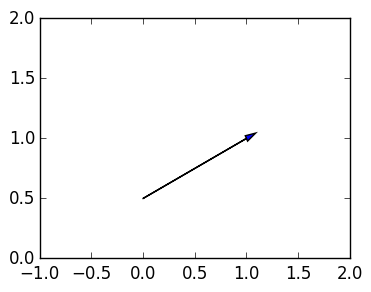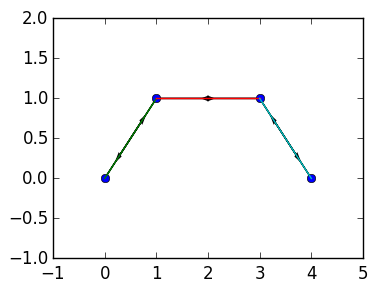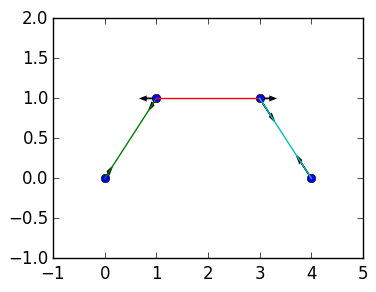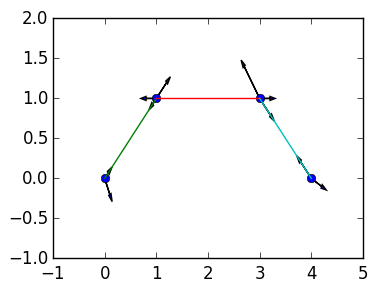# Linear system for structures¶

We return to the Structure example from Lab 3. In this lab we will construct a linear system that represents the forces from elongating bars, to determine whether or not a structure is stable.

Recall that the nodes are a sequence of $n$ points in 2D:

$$\mathbf{a}_1=(x_1,y_1),\mathbf{a}_2=(x_2,y_2),\ldots,\mathbf{a}_n=(x_n,y_n).$$

The bars are then given by a sequence of $m$ pairs of nodes:

$$\mathbf{b}_1=(p_1,q_1),\mathbf{b}_2=(p_2,p_2),\ldots,\mathbf{a}_n=(p_n,q_n),$$

where $p_j$ and $q_j$ are integers between 1 and $n$. For example, the bar attaching $\mathbf{a_2}$ to $\mathbf{a_4}$ is represented as $(1,4)$.

A Structure S has two fields: nodes, which are a $2 \times m$ matrix

$$S.nodes=\begin{pmatrix} x_1 & x_2 & \cdots & x_n \cr y_1 & y_2 & \cdots & y_n \end{pmatrix}$$

and bars, a $2 \times m$ matrix

$$S.bars=\begin{pmatrix} p_1 & p_2 & \cdots & p_n \cr q_1 & q_2 & \cdots & q_n \end{pmatrix}$$

## Setup code¶

Here is the necessary code from the last lab, that sets up a type called Structure:

In [ ]:
using PyPlot
import PyPlot.plot

type Structure
nodes::Matrix{Float64}
bars::Matrix{Int64}
end

function Structure(nodes::Matrix{Float64})
bars=zeros(Int,2,0)  # creates a 0 x 2 array
Structure(nodes,bars)
end

numnodes(S::Structure)=size(S.nodes,2)
numbars(S::Structure)=size(S.bars,2)

oldbars=S.bars
m=numbars(S)
newbars=zeros(2,m+1)

newbars[:,1:m]=oldbars
newbars[:,m+1]=[k,j]

S.bars=newbars  # replace S.bars with the newbars
S   # return S
end

function nodes(S::Structure,k)
S.nodes[:,S.bars[1,k]],S.nodes[:,S.bars[2,k]]
end

function plot(S::Structure)
A=S.nodes

# plot the nodes
x=vec(A[1,:])
y=vec(A[2,:])

plot(x,y;marker="o",linestyle="")

## TODO: plot bars
for k=1:numbars(S)
a,b=nodes(S,k)
plot([a,b],[a,b])
end

# the following code changes the axis to
minx=minimum(A[1,:])
maxx=maximum(A[1,:])

miny=minimum(A[2,:])
maxy=maximum(A[2,:])

axis([minx-1,maxx+1,miny-1,maxy+1])
end


## Normals¶

The force given by a bar is dictated by how elongated the bar is. But now we are living in 2D: the force is at an angle specified by the angle of the bar. This is given by $\mathbf{n}$ defined in terms of two nodes $\mathbf c$ and $\mathbf d$:

$$\mathbf{n}(\mathbf{c},\mathbf{d}) := {\mathbf{c} - \mathbf{d} \over \|\mathbf c - \mathbf d\|}$$

Exercise 1 What is $\mathbf{n}(\mathbf{c},\mathbf{d})$ corresponding to the bar connecting the node $\mathbf{c} = (0,0)$ to $\mathbf{d} = (1/\sqrt 2,1/\sqrt 2)$?

Thus a bar connecting $\mathbf{c}$ and $\mathbf{d}$ puts a force in the direction of $\mathbf{n}(\mathbf{c},\mathbf{d})$ on node $\mathbf{b}$ and in the direction of $-\mathbf{n}(\mathbf{c},\mathbf{d})$ on node $\mathbf{a}$: it is pulling each node in the opposite direction, towards each other.

We will represent the normal directions coresponding to a Structure by a $2 \times m$ matrix:

$$N:=\left(\mathbf{n}(\mathbf{a}_{p_1},\mathbf{a}_{q_1}) \ |\ \mathbf{n}(\mathbf{a}_{p_2},\mathbf{a}_{q_2})\ |\ \cdots\ |\ \mathbf{n}(\mathbf{a}_{p_m},\mathbf{a}_{q_m}) \right) = \left(\mathbf{n}_1\ |\ \mathbf{n}_2\ |\ \cdots\ |\ \mathbf{n}_m \right) = \begin{pmatrix} n_1^x & n_2^x & \cdots & n_m^x \cr n_1^y & n_2^y & \cdots & n_m^y \end{pmatrix}$$

Exercise 2(a) Complete the following function that returns the normal matrix for a given structure:

In [ ]:
normal(a,b)=(a-b)/norm(a-b)
function normals(S::Structure)
N=zeros(Float64,2,numbars(S))

for k=1:numbars(S)
a,b=nodes(S,k)  # remember: nodes(S,k) gives the two nodes connected to the k-th bar
# TODO: populate N
end
N
end


2(b) What should the following return? Does the output match what you expected?

In [ ]:
S=Structure([0. 1. 3. 4.;
0. 1. 1. 0.],
[1 2 3;
2 3 4])

normals(S)


## Plotting arrows¶

The arrow(x,y,δx,δy) command in PyPlot plots an arrow from the point (x,y) to (x+δx,y+δy), where the arrow head appears after the line. The optional arguments head_width and head_length can be used to set the size and shape of the arrow:

In :
arrow(0.,0.5,1.,0.5; head_width=0.05,head_length=0.1)
axis([-1,2,0,2]);Exercise 3(a) Complete the following function that, for each bar, plot two arrows depicting the direction of the force on each node. See the successful plot below as an example:

In :
function plotnormals(S::Structure)
N=normals(S)

# TODO: for each bar, plot two arrows in the direction on the forces on the connecting nodes
end;


3(b) Ensure your plotnormals routine plots the following figure correctly:

In :
S=Structure([0. 1. 3. 4.;
0. 1. 1. 0.],
[1 2 3;
2 3 4])

plot(S)
plotnormals(S)## Forces from bars¶

$\mathbf n$ only indicate the direction in which the bars will put forces on the structure. The magnitude will be given by Hooke's law: if the bar $\mathbf{b}_k$ is elongated by an amount $e_k$, then the force will be of magnitude $y_k = c_k e_k$, where $c_k$ is the stiffness of the bar. We will take $c_k = 1$, and assume that a given Structure represents the bars at rest.

Therefore, elongations will be represented by a a vector of length $m$, one for each bar: $$\mathbf{e} = \begin{pmatrix} e_1 \cr e_2 \cr \vdots \cr e_m\end{pmatrix}$$

The direction of the force is given by $N$ above, that is, the force is $\mathbf y_k$ on node $\mathbf a_{q_k}$ and $-\mathbf y_k$ on node $\mathbf a_{p_k}$, where

$$\mathbf y_k = e_k \mathbf n_k.$$

Exercise 4(a) Complete the following function that plots arrows representing the forces of each bar: this time, the length of the arrow is given by $e_k$. (Hint: Copy-and-paste and modify plotnormals.)

In [ ]:
function plotbarforces(S::Structure,e::Vector{Float64})
#TODO: For each bar, plot two normals showing the forces specified by the vector e
end;


4(b) Check that the following picture is plotted correctly, where the first and third bar are stretched while the second bar is squeezed:

In :
S=Structure([0. 1. 3. 4.;
0. 1. 1. 0.],
[1 2 3;
2 3 4])

plot(S)
plotbarforces(S,[0.1,-0.2,0.3])4(c) Can you write a one-line redefinition of plotnormals by calling plotbarforces?

In [ ]:



## External forces¶

The external forces will be given by vectors $\mathbf{f}_1$, $\mathbf{f}_2$, …, $\mathbf{f}_n$, one external force per node. They will be represented by the $2 \times n$ matrix

$$F := \left(\mathbf f_1\ |\ \cdots\ |\ \mathbf f_n \right) = \begin{pmatrix} f_1^x & f_2^x & \cdots & f_n^x \cr f_1^y & f_2^y & \cdots & f_n^y \end{pmatrix}$$

Exercise 5(a) Complete the following function that plots the external forces given by the $2 \times n$ matrix F, eminating from the $k$th node of S. (Hint: recall that S.nodes[:,k] gives the $x$ and $y$ coordinates of the kth node.)

In [ ]:
function plotexternalforces(S::Structure,F::Matrix)
# TODO: plot an arrow eminating from node a in the direction specified by the k-th column of F
end;


5(b) Ensure that the following is plotted correctly:

In :
S=Structure([0. 1. 3. 4.;
0. 1. 1. 0.],
[1 2 3;
2 3 4])

plotbarforces(S,[0.1,-0.2,0.3])
plotexternalforces(S,[.1 .2 -.3 .2;  -0.2 0.2 .4 -.1])
plot(S);## Displacements¶

We want to model the displacement $\mathbf{u}_k$ of each node $\mathbf{a}_k$ under forces, or as a matrix

$$U =\left(\mathbf u_1\ | \ \cdots \ |\ \mathbf u_n \right)= \begin{pmatrix} u_1^x & \cdots & u_n^x \cr u_1^y & \cdots &u_n^y \end{pmatrix}$$

Now consider how far the bars are elongated. The true elongation is the length of the new bar, which is found by finding the difference between the two nodes:

$$e_k^{\rm true} = \|\mathbf{b}_{p_k} - \mathbf{b}_{q_k}\|$$

where $k=1,\ldots,m$. This leads to a nonlinear equation, unfortunately.

We assume that the displacements are small, and so we can replace the true elongation with a linear model. We will use

$$e_k = \mathbf{n}_k^\top (\mathbf{u}_{p_k} - \mathbf{u}_{q_k}) \qquad \hbox{for} \qquad \mathbf{n}_k := {\mathbf{a}_{p_k} - \mathbf{a}_{q_k} \over \|\mathbf a_{p_k} - \mathbf{a}_{q_k}\|}$$

We want to convert this equation giving $e_k$ in terms of $U$ into an equation. We first construct two $m \times n$ matrices, $M_x$ and $M_y$ so that

$$\mathbf e = M_x \begin{pmatrix} u_1^x \cr \vdots \cr u_n^x \end{pmatrix} + M_y \begin{pmatrix} u_1^x \cr \vdots \cr u_n^x \end{pmatrix}$$

Exercise 6(a) Why are these matrices given by the property that

$$M_x[k,p_k] = n_k^x$$$$M_x[k,q_k] = -n_k^x$$

$$M_y[k,p_k] = n_k^y$$ $$M_y[k,q_k] = -n_k^y$$

with zero entries otherwise, where $M_x[k,j]$ denotes the $(k,j)$ entry of $M_x$?

6(b) Complete the following routine that returns two matrices Mx and My that give $M_x$ and $M_y$ as defined above:

In :
function incidentmatrices(S::Structure)
m=numbars(S)
n=numnodes(S)
B=S.bars

Mx=zeros(m,n)
My=zeros(m,n)
N=normals(S)
for k=1:m   # populate e_k
# TODO: add the appropriate entries to Mx and My
end

Mx,My
end;


6(c) Ensure that Mx returns the correct value here:

In :
S=Structure([0. 1. 3. 4.;
0. 1. 1. 0.],
[1 2 3;
2 3 4])

Mx,My=incidentmatrices(S)
Mx

Out:
3x4 Array{Float64,2}:
-0.707107   0.707107   0.0       0.0
0.0       -1.0        1.0       0.0
0.0        0.0       -0.707107  0.707107

6(d) Without evaluating it, predict what My is. Are you correct?

In [ ]:
My


## Reducing a matrix of unknowns to a vector of unkowns¶

Are definition

$$\mathbf e = M_x \begin{pmatrix} u_1^x \cr \vdots \cr u_n^x \end{pmatrix} + M_y \begin{pmatrix} u_1^x \cr \vdots \cr u_n^x \end{pmatrix}$$

is not in the correct form for a linear system. We want to reduce it to a single vector of unknowns:

$$\mathbf u = \begin{pmatrix} u_1^x \cr u_1^y \cr u_2^x \cr u_2^y \cr \vdots \cr u_n^x \cr u_n^y \end{pmatrix}$$

We then combine $M_x$ and $M_y$ into a single $m \times 2n$ matrix:

$$\mathbf{e} = M \mathbf u$$

by interlacing:

$$M = \begin{pmatrix} M_x[1,1] & M_y[1,1] & M_x[1,2] & M_y[1,2] & \cdots & M_x[1,n] & M_y[1,n] \cr \vdots & \vdots & \vdots & \vdots & \ddots & \vdots & \vdots \cr M_x[m,1] & M_y[m,1] & M_x[m,2] & M_y[m,2] & \cdots & M_x[m,n] & M_y[m,n] \end{pmatrix}$$

Exercise 7(a) What Julia function reduces a Matrix $U$ to the Vector $\mathbf u$?

7(b) Complete the following function that combines Mx and My to form a single matrix M:

In :
function incidentmatrix(S::Structure)
Mx,My=incidentmatrices(S)

M=zeros(size(Mx,1),2size(Mx,2))
# TODO: Populate the entries of M

M
end;


7(c) Check the following:

In :
M=incidentmatrix(S)
U=[.1 .2 -.3 .2;  -0.2 0.2 .4 -.1]
M*vec(U)

Out:
3-element Array{Float64,1}:
0.353553
-0.5
0.707107

7(d) Use plotbarforces along with M above to plot the forces given by $U$. What happens if $U$ is changed to $0.01U$?

In [ ]:



## Forces sum to zero¶

goal is to find displacements $\mathbf{u}_k$ so the forces on each node — the arrows in the above picture! — sum to zero: on node $k$, we want

$$0 = \mathbf{f}_k + \sum_{j=1}^m \begin{cases} -y_j \mathbf{n}_j & if p_j = k \cr y_j \mathbf{n}_j & if q_j = k \cr 0 & otherwise \end{cases}$$

Solving this will be the topic of the next lab. If you want to jump ahead, consider the following two questions:

Exercise 8(a) (Advanced) What is the equation relating $y_j$ and $vec(F)$, in terms of the matrix $M$?

8(b) (Advanced) Evaluate nullspace(M'*M). What issue does this raise about the problem we are trying to solve?

In [ ]:
nullspace(M'*M)